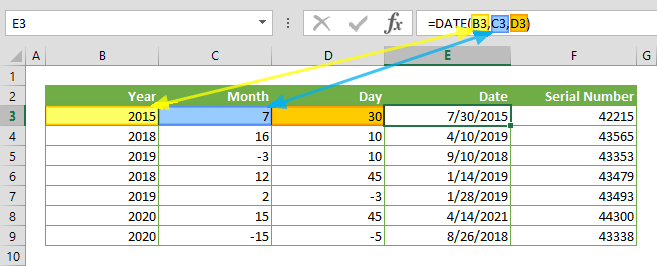The DATE function in Excel returns a date serial number from specified year, month and day values. In this guide, we’re going to show you how to use the DATE function and also throw in some tips and error handling methods.

# Supported versions

• All Excel versions

# Date Function in Excel Syntax

DATE(year,month,day)

# Arguments

 year An integer from 0 to 9999 representing year. 0 is equal to year 1900. month A positive or negative integer representing the month of the year from 1 to 12. day A positive or negative integer representing the day of the month from 1 to 31.

Example

=DATE(C3,D3,E3)
formula returns a serial number for the date 7/30/2015 for year 2015, month 7 and day 30.# Tips

• Excel stores dates as numbers. Excel assumes that the date 1/1/1900 is "1", and increases this number by 1 for each day. For example; 12/31/2018 is equal to 43465. Because of this, the DATE function in Excel returns a number that is displayed as a date.
• Prefer using 4-digit years to prevent confusion. For example; 18 can mean either 1918 and 2018.
• If month is greater than 12, Excel adds months to the first month of the specified year. For example;

=DATE(2018,16,10)

returns the date April 10, 2019.

• If month is less than 1, Excel subtracts the absolute value of the month from the first month of the specified year. For example;

=DATE(2019,-3,10)

returns September 9, 2018.

• If day is greater than 31, Excel adds days to the first day of the specified month. For example;

=DATE(2018,12,45)

returns the date January 14, 2019.

• I day is less than 1, Excel subtracts the absolute value of the day from the first day of the specified month. For example;

=DATE(2018,2,-3)

returns January 28, 2019.

• The DATEVALUE function can be used to convert date strings into Excel's number system.

# Issues

## #NUM!

• If the year value is less than 0 or greater than 9999, you will get the #NUM! error.